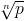# 程序控

IPPP (Institute of Penniless Peasent-Programmer) Fellow

:: :: :: :: :: :: :: ::77 随笔 :: 0 文章 :: 442 评论 :: 0 引用

Time limit: 3.000 seconds

## Background背景

Current work in cryptography involves (among other things) large prime numbers and computing powers of numbers modulo functions of these primes. Work in this area has resulted in the practical use of results from number theory and other branches of mathematics once considered to be of only theoretical interest.

This problem involves the efficient computation of integer roots of numbers.

## The Problem问题

Given an integer n ≥ 1 and an integer p ≥ 1 you are to write a program that determines, the positive nth root of p. In this problem, given such integers n and p, p will always be of the form kn for an integer k (this integer is what your program must find).

## The Input输入

The input consists of a sequence of integer pairs n and p with each integer on a line by itself. For all such pairs 1 ≤ n ≤ 200, 1 ≤ p ≤ 10101and there exists an integer k, 1 ≤ k ≤ 109 such that kn = p.

## The Output输出

For each integer pair n and p the valueshould be printed, i.e., the number k such that kn = p.

## Sample Input

2
16
3
27
7
4357186184021382204544

4
3
1234

## Solution

#include <iostream>
#include <math.h>
using namespace std;
int main(void) {
for (double dNum, dPow; cin >> dPow >> dNum;
cout << (int)(pow(dNum, 1.0 / dPow) + 0.5) << endl);
return 0;
}

posted on 2010-08-10 22:22  Devymex  阅读(...)  评论(...编辑  收藏